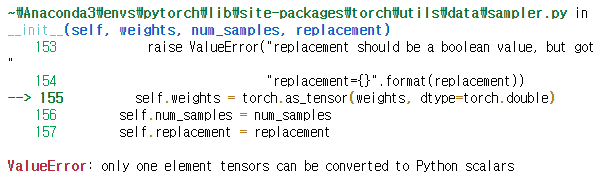# Unbalanced Data, Overfitting occurs

I am currently working on binary segmentation.

When using unbalance data, overfitting occurs.
Below is a loss curve.I am using BCEWithLogitsLoss, and pos_weight is applied by calculating neg/positive.

The distribution of data has a ratio [data without segments: data with segments] = about 20:1

The learning rate varied from 1e-3 to 1e-7, and weight_decay applied 1e-4 and dropout.

I tried applying the various methods found on the pytorch forum, but the result is not improved.
I think it’s because the data is too unbalanced,
How can I solve it?

Did you check the confusion matrix for the original loss function and the one using `pos_weight` and did something change?
I would recommend to use unreasonably large or small values for the weighting and make sure that your training achieves the desired effect. You could also try to use `WeightedRandomSampler` for over-/undersampling.

I am applying pos_weight by counting the number of negative and positive numbers as shown below.

As a difference between before and after applying pos_weight, I was confirmed whether training loss (+dice) was reduced.

``````        data_loader = torch.utils.data.DataLoader(traindataset, batch_size = batch_size, shuffle=True, num_workers=0, pin_memory=False)

pos = 0.0
neg = 0.0
for p in range(len(y)):
if y[p].max()==1:
pos+=1
else:
neg+=1
weights = neg/pos
pos_weight = torch.tensor(weights)

for batch_idx , (data,target) in enumerate(data_loader):
inputs,target = data.to(device),target.to(device)
...
criterion = nn.BCEWithLogitsLoss(reduction='mean', pos_weight=pos_weight)
``````

Should I set the value of pos_weight randomly without calculating like this?

The confusing part of this sampler is when there is llabel(value have 1) and no label(value only 0) (i.e. binary problem),
`class_sample_count = []` What value should I put in this part?

`class_sample_count = [positive_count]` ? or
`class_sample_count = [negative_count,positive_count]`?

Yes, for the same of debugging try out different values and check the confusion matrix to verify that the predictions really change.

You should use both classes to calculate the class count and assign the computed weight to each sample.

1. I tried the following debugging in the same setting.

1). pos_weight <0; 0.2 scale
2). pos_weight = None
3). pos_weight >= 1; scale 10 1 to 100

As a result,
In 1) confirmed that the loss value decreased similarly for both train and valid, but it was confirmed that the confusion matrix and dice values did not improve each epoch.

In 2), I saw a lot of changes in both train loss and valid loss.

In 3), similar to the graph in the question, train loss decreased well, but valid loss changed significantly.

I think the 3) case is appropriate, but I wonder if there are other factors that reduce the fluctuation of valid?

1. I configured the code as below to use `WeightedRandomSampler`, but I am not sure how to load the target because I am using `concat dataset`.
``````        train_datasets = []
for i in range(5):
secure_random = random.SystemRandom()
random_patient = secure_random.choice(patient_index)
train_datasets.append(trainDataset(random_patient,"data_path",augmentation=True))
patient_index.remove(random_patient)
traindataset = torch.utils.data.ConcatDataset(train_datasets)

class_sample_count = [neg,pos]  # I already calculated negative/positive count
weights = 1/torch.tensor(class_sample_count, dtype=torch.float)
samples_weights = [weights[t] for t in target]
sampler = torch.utils.data.sampler.WeightedRandomSampler(weights=samples_weights, num_samples=len(samples_weights, replacement=True)
``````

Is there a way to load the target in this code `samples_weights = [weights[t] for t in target]`?

My custom dataset (=`trainDataset`) follows the structure below.

``````class trainDataset(torch.utils.data.Dataset):
def __init__(self, i, data_path, augmentation=True):
self.data_path = data_path
self.data = self.data-self.data.mean()
self.data = self.data/self.data.std()
self.augmentation = augmentation
def __getitem__(self, index):
x = self.data[index]
y = self.target[index]
x, y = self.transform(x, y)

return x, y

def transform(self, data, target):
data, target = data_augmentation(data, target, self.augmentation)
return data, target

def __len__(self):
return len(self.data)
``````
1. In my think, if I use `WeightedRandomSampler`, use of `pos_weight` doesn’t seem to make sense. Is this correct?

Thanks!

Did your model predictions change from the negative to the positive class or vice verse when you’ve changed the `pos_weight`?

I don’t think a negative `pos_weight` would make sense, would it?

If you are creating the targets lazily in each iteration, you could create the target tensor once before the training via:

``````targets = []
for _, target in dataset:
targets.append(target)
targets = torch.stack(targets)
``````

and use it to create the `WeightedRandomSampler`.

Might be the case, but I would recommend to run experiments and verify that each step is working as expected.

Oh, I wrote the wrong thing about 1). Correct with `0<pos_weight <1`.

The model’s prediction changes from 0. to <1 unless the value of pos_weight is less than 1.

Here is an example of learning when pos_weight is greater than 1.As you can see from the above valid loss value, there was a change in the value that increased and decreased at a certain moment.

In addition, when Icreate targets using the method you informed, I get the following error.Is it wrong to create targets and weight each sample with `samples_weights = [weights[t] for t in targets]`?

Thanks!